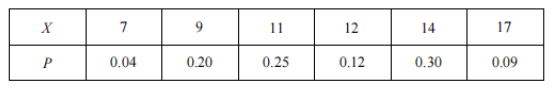(1) 求 $X=7$ 的概率;
(2) 已知考生甲第 9 题全部选对, 第 10 题部分选对, 求随机变量 $X$ 的分布列与期望.
【答案】 解: (1) $P(X=7)=\mathrm{C}_4^2 \times 0.2^2 \times \mathrm{C}_2^1 \times 0.5 \times 0.3=0.072$.
(2) $X$ 的所有可能取值为 $7,9,11,12,14,17$, 则
\begin{aligned} & P(X=7)=\mathrm{C}_2^2 \times 0.2^2=0.04, \quad P(X=9)=\mathrm{C}_2^1 \times 0.2 \times 0.5=0.20, \\ & P(X=11)=\mathrm{C}_2^2 \times 0.5^2=0.25, \quad P(X=12)=\mathrm{C}_2^1 \times 0.2 \times 0.3=0.12, \end{aligned}

$P(X=14)=\mathrm{C}_2^1 \times 0.5 \times 0.3=0.30, \quad P(X=17)=\mathrm{C}_2^2 \times 0.3^2=0.09$$E(X)=0.28+1.8+2.75+1.44+4.20+1.53=12$